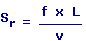Strouhal Number Calculator Enter value, select unit and click on calculate. Result will be displayed.Sr = Strouhal Number V = Velocity L = Characteristic Length f = Frequency
 Enter your values: Characteristic Length (L): Meter Centimeter Attometer Dekameter Decimeter Exameter Femtometer Foot Hectometer Inch Kilometer Megameter Micrometer Mile Millimeter Nanometer Petameter Picometer Yard Frequency (f): Hertz Kilohertz Megahertz Gigahertz Velocity (v): Meter / Second Foot / Second Centimeter / Hour Centimeter / Second Foot / Day Inch / Hour Inch / Second Kilometer / Second Knot Meter / Day Mile / Hour Mile / Second Millimeter / Second Yard / Second Result: Strouhal Number (Sr):

 Web www.calculatoredge.com
 Strouhal number is a dimensionless number used to describe oscillating flow mechanisms. It is named after Vincenc Strouhal.

The Strouhal Number is important for analyzing unsteady, oscillating flow problems. The Strouhal Number represents the ratio of inertial forces due to the unsteadiness of the flow or local acceleration to the inertial forces due to changes in velocity from one point to an other in the flow field.LINKSDISCLAIMERCONTACT US